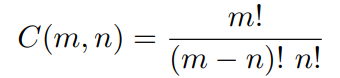TORONTO KIDS COMPUTER CLUB | Aurora Saturday 14:30 Python Practice 22.11.19.
21424

# Aurora Saturday 14:30 Python Practice 22.11.19.

## 23 Nov Aurora Saturday 14:30 Python Practice 22.11.19.

Question:

The binomial coefficient C(m, n) is defined asGiven four natural numbers p, q, r, and s, compute the the result of dividing C(p, q) by C(r, s).

Input
Input consists of a sequence of lines. Each line contains four non-negative integer numbers giving values
for p, q, r, and s, respectively, separated by a single space. All the numbers will be smaller than 10,000
with p ≥ q and r ≥ s.

Output
For each line of input, print a single line containing a real number with 5 digits of precision in the fraction, giving the number as described above. You may assume the result is not greater than 100,000,000.

`Sample Input10 5 14 993 45 84 59145 95 143 92995 487 996 4882000 1000 1999 9999998 4999 9996 4998Sample Output0.12587505606.460551.282230.489962.000003.99960`